Скачать презентацию Generation and control of highorder harmonics by the

2c135d4925be765a29d79aa7be9802bc.ppt

• Количество слайдов: 33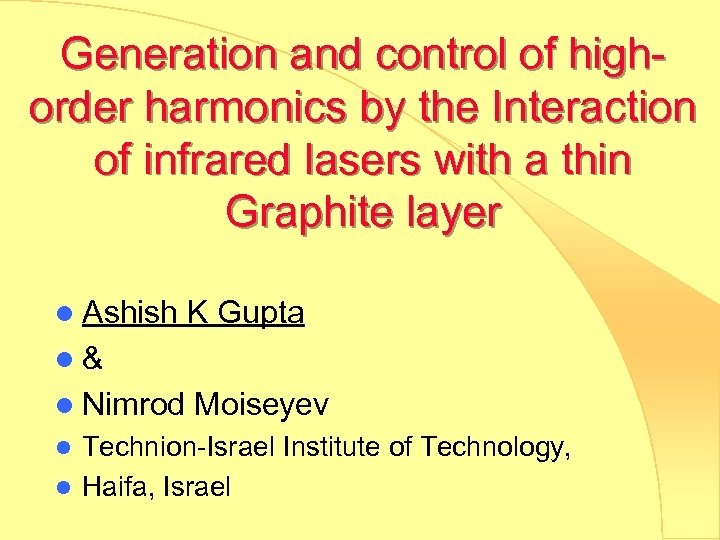Generation and control of highorder harmonics by the Interaction of infrared lasers with a thin Graphite layer l Ashish K Gupta l& l Nimrod Moiseyev Technion-Israel Institute of Technology, l Haifa, Israel l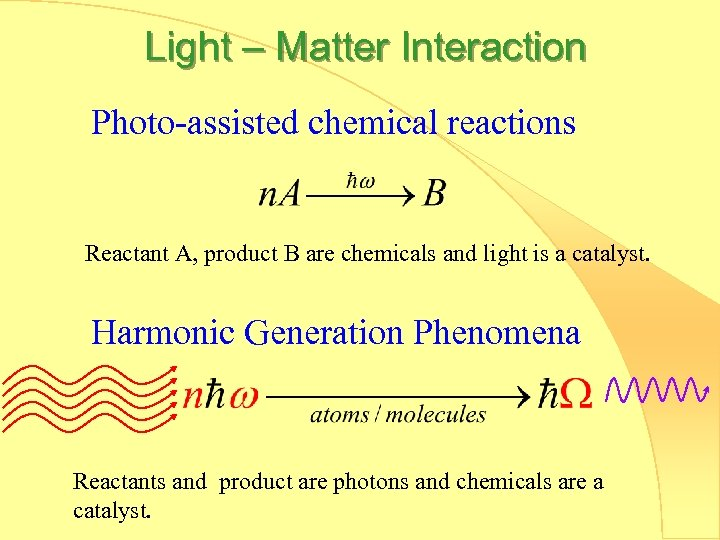Light – Matter Interaction Photo-assisted chemical reactions Reactant A, product B are chemicals and light is a catalyst. Harmonic Generation Phenomena Reactants and product are photons and chemicals are a catalyst.Mechanism for generation of high energy photons (high order harmonics) Multi-photon absorption ħω k E z Radiation ħΩ Acceleration of electron Probability to get high energy photon ħΩ ħω:Quantum-mechanical solution Time-dependent wave-function of electron (t) Acceleration of electron Hamiltonian with electron-laser interaction Linearly Polarized light: Circularly Polarized light: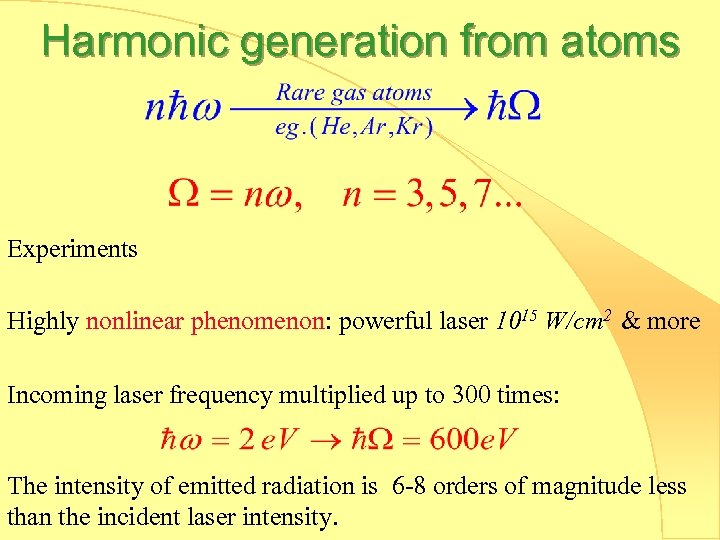Harmonic generation from atoms Experiments Highly nonlinear phenomenon: powerful laser 1015 W/cm 2 & more Incoming laser frequency multiplied up to 300 times: The intensity of emitted radiation is 6 -8 orders of magnitude less than the incident laser intensity.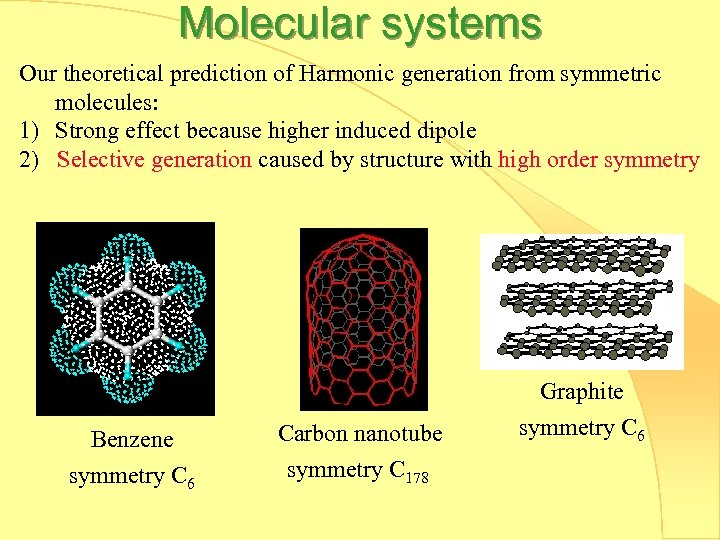Molecular systems Our theoretical prediction of Harmonic generation from symmetric molecules: 1) Strong effect because higher induced dipole 2) Selective generation caused by structure with high order symmetry Graphite Benzene symmetry C 6 Carbon nanotube symmetry C 178 symmetry C 6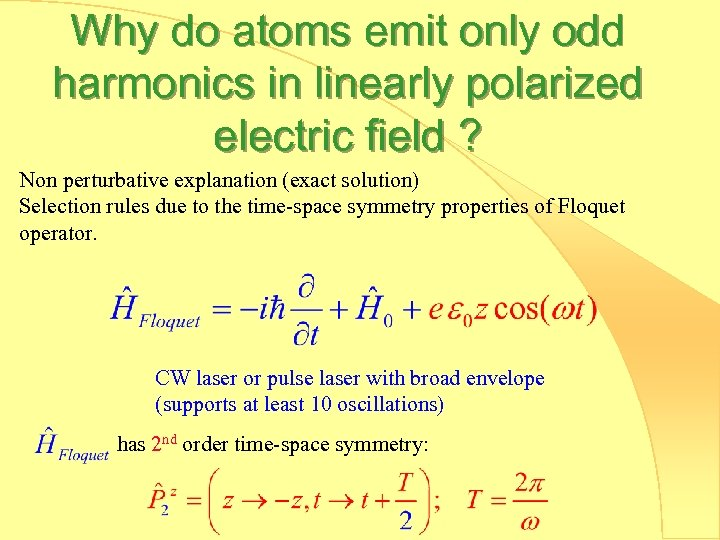Why do atoms emit only odd harmonics in linearly polarized electric field ? Non perturbative explanation (exact solution) Selection rules due to the time-space symmetry properties of Floquet operator. CW laser or pulse laser with broad envelope (supports at least 10 oscillations) has 2 nd order time-space symmetry: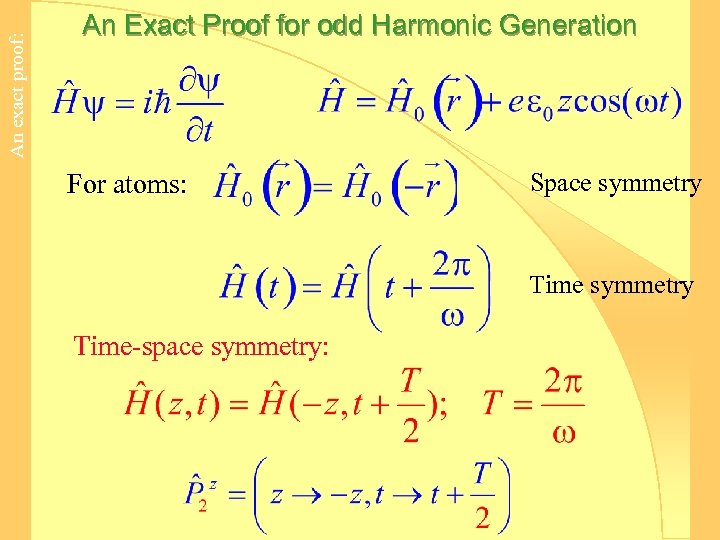An exact proof: An Exact Proof for odd Harmonic Generation For atoms: Space symmetry Time-space symmetry: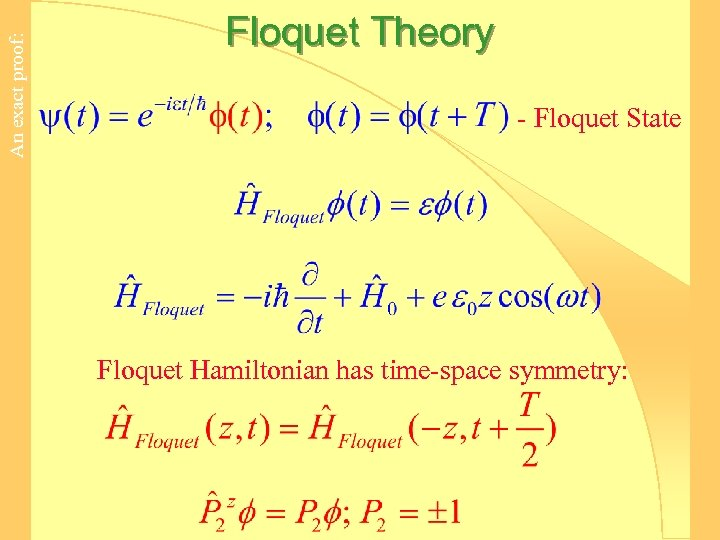An exact proof: Floquet Theory - Floquet State Floquet Hamiltonian has time-space symmetry: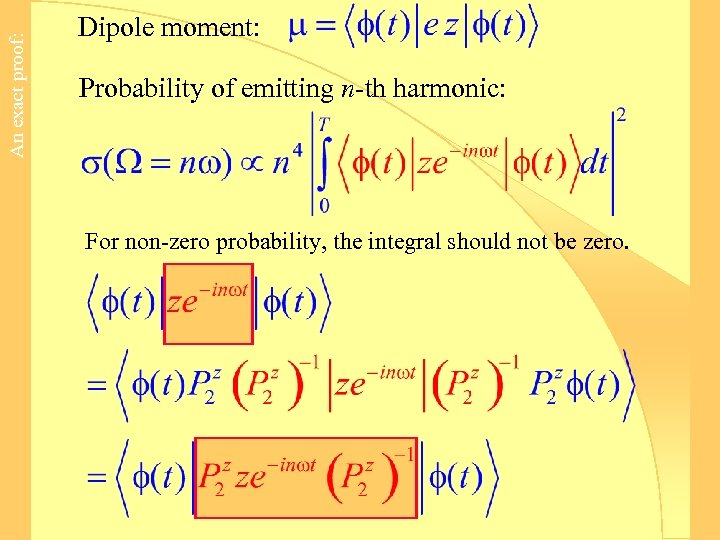An exact proof: Dipole moment: Probability of emitting n-th harmonic: For non-zero probability, the integral should not be zero.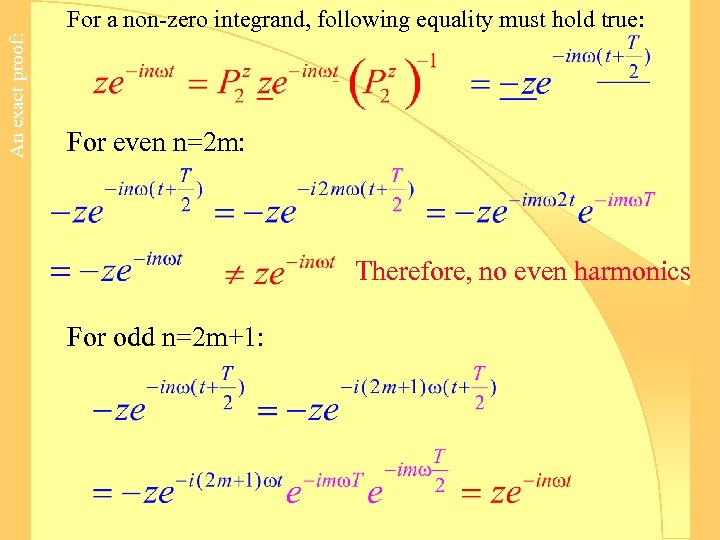An exact proof: For a non-zero integrand, following equality must hold true: For even n=2 m: Therefore, no even harmonics For odd n=2 m+1: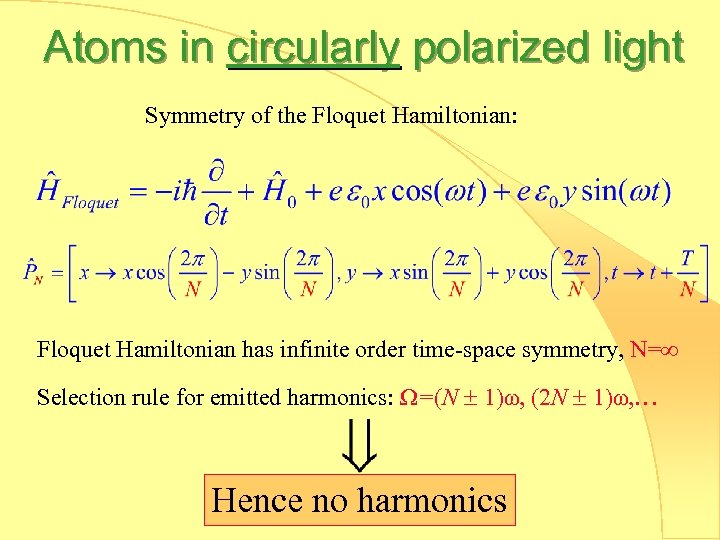Atoms in circularly polarized light Symmetry of the Floquet Hamiltonian: Floquet Hamiltonian has infinite order time-space symmetry, N= Selection rule for emitted harmonics: Ω=(N 1)ω, (2 N 1)ω, … Hence no harmonics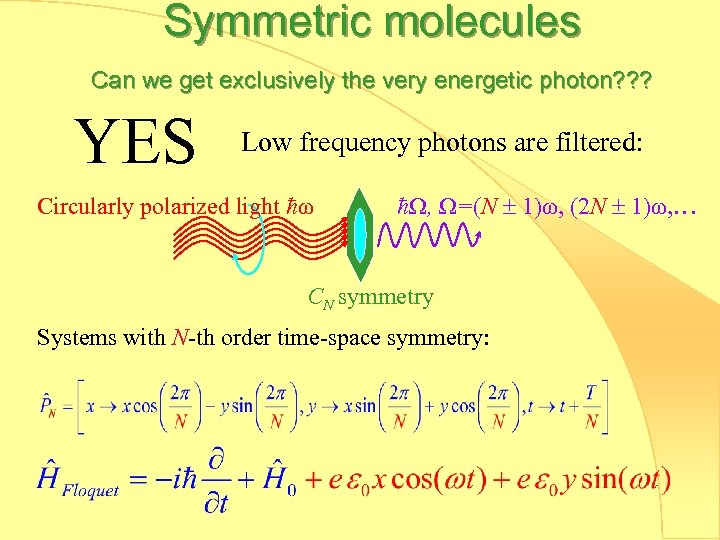Symmetric molecules Can we get exclusively the very energetic photon? ? ? YES Low frequency photons are filtered: Circularly polarized light ħω ħΩ, Ω=(N 1)ω, (2 N 1)ω, … CN symmetry Systems with N-th order time-space symmetry:Graphite C 6 symmetry (6 th order time-space symmetry in circularly polarized light) Numerical Method: 1) Choose the convenient unit cell 2) Tight binding basis set 3) Bloch theory for periodic solid structure 4) Floquet operator for description of time periodic system 5) Propagate Floquet states with time-dependent Schrödinger equation.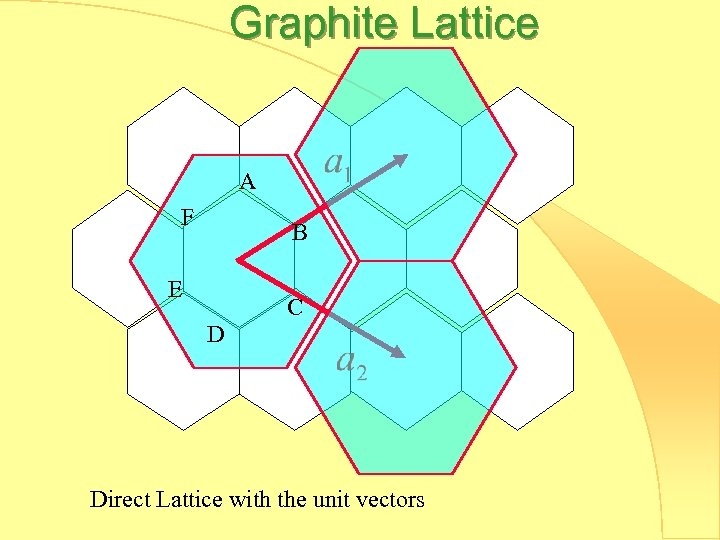Graphite Lattice A F B E C D Direct Lattice with the unit vectors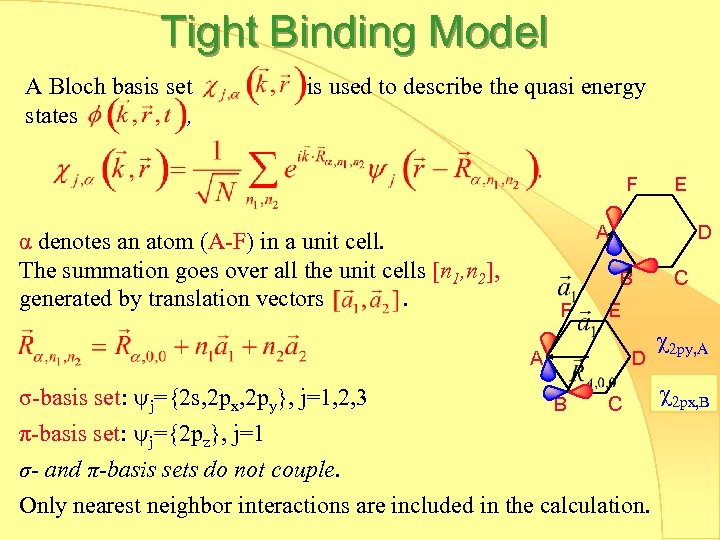Tight Binding Model A Bloch basis set states , is used to describe the quasi energy F E A α denotes an atom (A-F) in a unit cell. The summation goes over all the unit cells [n 1, n 2], generated by translation vectors. D B F A C E D 2 py, A 2 px, B σ-basis set: j={2 s, 2 px, 2 py}, j=1, 2, 3 B C π-basis set: j={2 pz}, j=1 σ- and π-basis sets do not couple. Only nearest neighbor interactions are included in the calculation.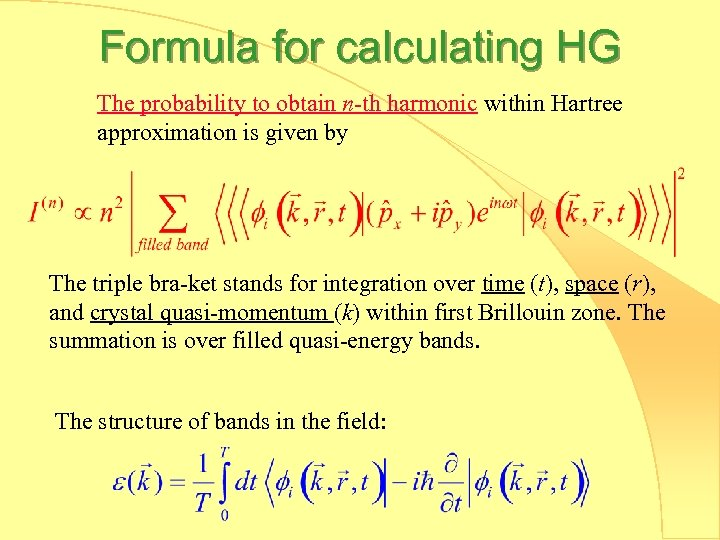Formula for calculating HG The probability to obtain n-th harmonic within Hartree approximation is given by The triple bra-ket stands for integration over time (t), space (r), and crystal quasi-momentum (k) within first Brillouin zone. The summation is over filled quasi-energy bands. The structure of bands in the field: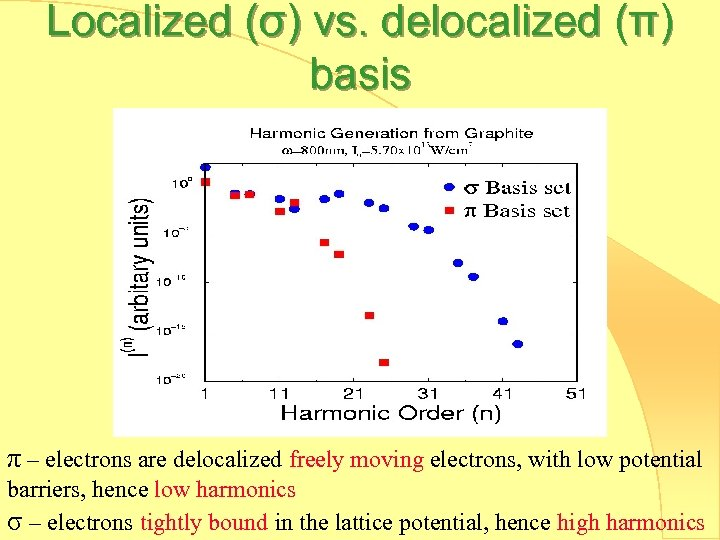Localized (σ) vs. delocalized (π) basis π – electrons are delocalized freely moving electrons, with low potential barriers, hence low harmonics σ – electrons tightly bound in the lattice potential, hence high harmonics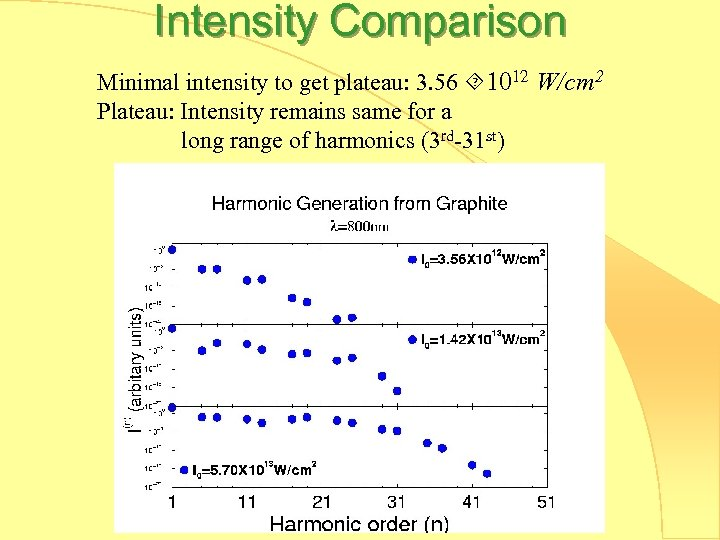Intensity Comparison Minimal intensity to get plateau: 3. 56 1012 W/cm 2 Plateau: Intensity remains same for a long range of harmonics (3 rd-31 st)Effect of laser frequency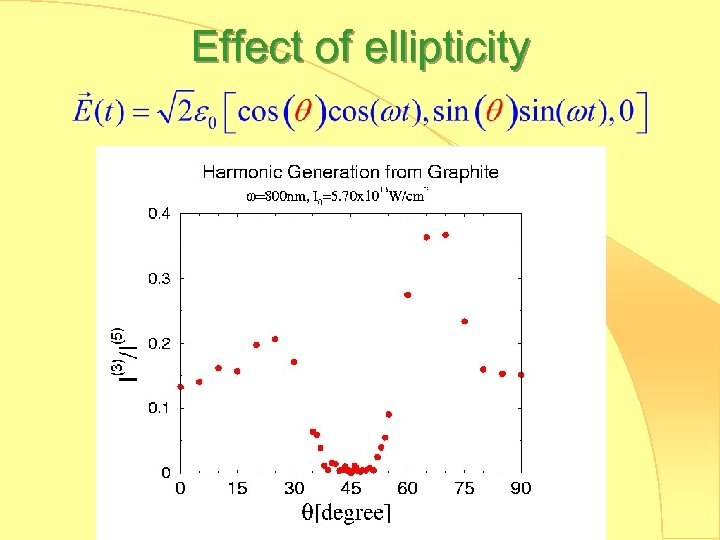Effect of ellipticityGraphite vs. Benzene HG from Benzene-like structure dies faster than HG from Graphite. No enhancement of the intensity using circularly vs. linearly polarized light is obtained, Hence it is a filter, not an amplifier.Conclusions 1. High harmonics predicted from graphite. 2. Interaction of CN symmetry molecules/materials with circularly polarized light rather than with linearly polarized light, generates photons with energy ħΩ where Ω=(N 1)ω, (2 N 1)ω, … 3. Circularly polarized light filters the low energy photons, however no amplification effect is predicted. 4. Extended structure produces longer plateau as seen in the case of Graphite vs. benzene-like systems. 5. HG in graphite is stable to distortion of symmetry. For 1% distortion of the polarization the intensity of the emitted 5 th (symmetry allowed) harmonic is 100 times larger than the intensity of the 3 rd (forbidden) harmonic.Thanks Prof. Nimrod Moiseyev Prof. Lorenz Cederbaum Dr. Ofir Alon Dr. Vitali Averbukh Dr. Petra Žďánská Dr. Amitay Zohar Aly Kaufman Fellowship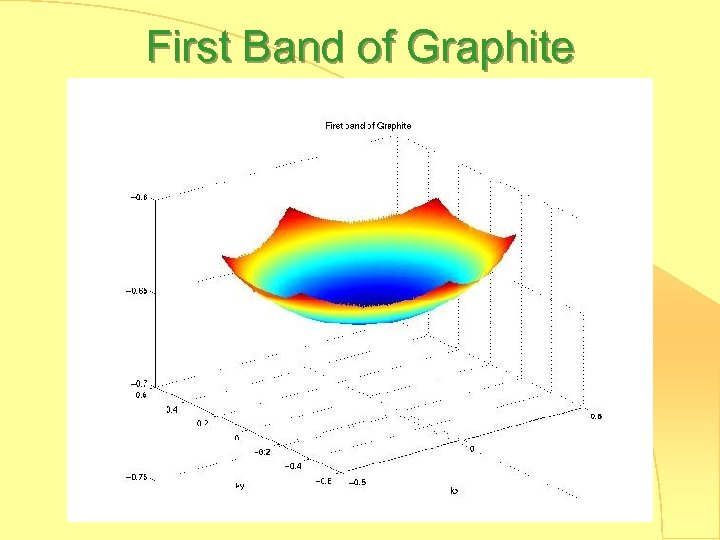First Band of GraphiteHG due to acceleration in xHG due to acceleration in y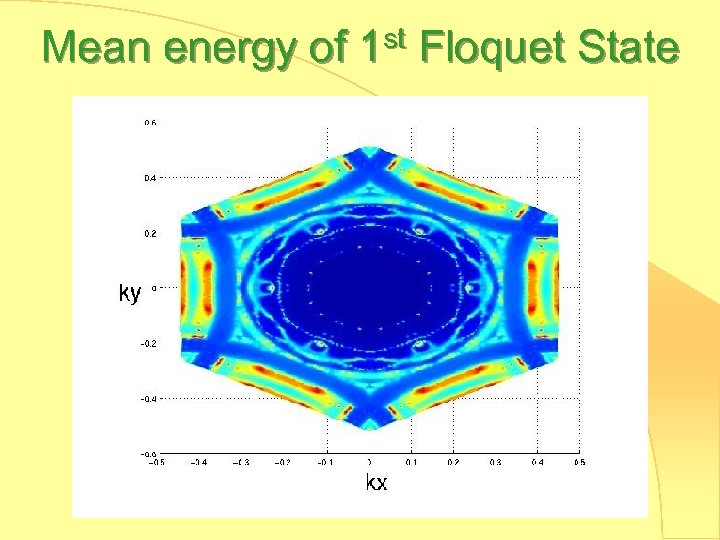Mean energy of 1 st Floquet StateFirst quasi energy bandAvoided crossing for 1 st Floquet StateEntropy of 1 st Floquet State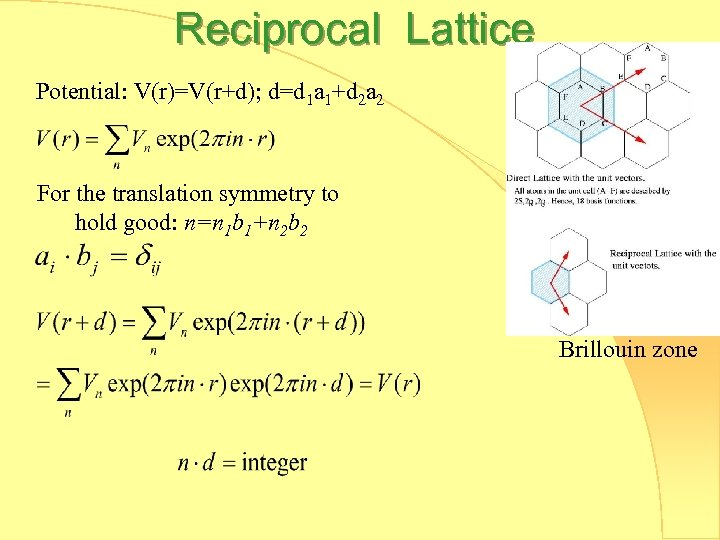Reciprocal Lattice Potential: V(r)=V(r+d); d=d 1 a 1+d 2 a 2 For the translation symmetry to hold good: n=n 1 b 1+n 2 b 2 b 1 b 2 Reciprocal lattice: Brillouin zone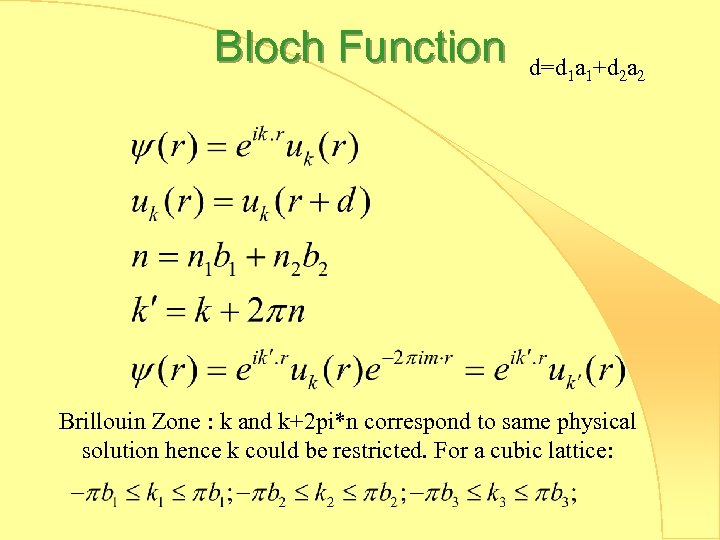Bloch Function d=d 1 a 1+d 2 a 2 Brillouin Zone : k and k+2 pi*n correspond to same physical solution hence k could be restricted. For a cubic lattice: